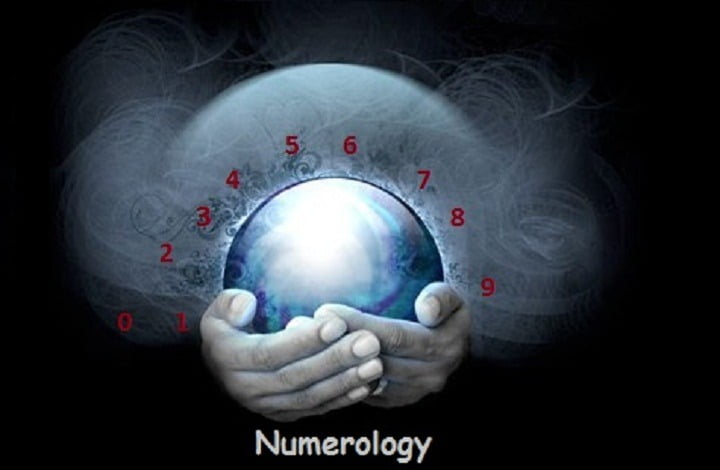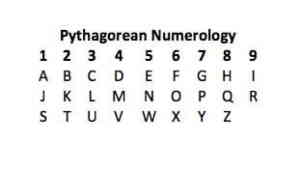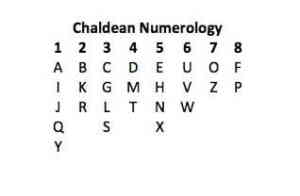# AstroKapoor### Basic of Numerology

Basic of Numerology discusses about the numbers and the role of numbers in astrological science. Numerology is a branch of science which deals with connections between numbers and personality traits, events, and circumstances. This can also be called as branch of divination similar to astrology, and there is also a belief that certain numbers are associated with people, places, and events and may play a role in understanding these things better.

## Types of Numerology

Numerology comes from various origins, and different people use different types.

### Gematria

Gemtaria system is a branch of numerology which arise from jewish mystician and scripture interpretation where each Hebrew letter is assigned a numerical value and the value of words were calculated using these numbers. Using this equivalence, scholars calculated the value of words in the scriptures.

Get your numerology report

#### Pythagorean Numerology

This was used by Western system and can be traced back to Greek philosopher and mathematician Pythagoras. He developed numbers. In Gemtaria, Pythagoras placed numbers offered a system that understands the universe, and he also assigned each letter of the alphabet a numeric value. This is the most common form of numerology practiced today.In Pythagorean system, one calculates the numerical value which is important, such as name and then reduces it to a single numeric value using the following values of letters.

##### Chaldean Numerology

Chaldean system can be dated back older than Pythagorean system and was developed by the Chaldeans. This is based on eight numerals, and many people find it difficult to master more accurate than the more commonly used Pythagorean system.Like Pythagorean numerology, Chaldean system gives differing values to letters of the alphabet as indicated in the chart below.

##### Kabbalah Numerology

Kabbalah system uses the name to calculate personality traits in a manner similar to the Pythagorean system but it was with different meanings for each single digit numbers. Kabbalah has more than 400 life path combinations, so there are more variables in Kabbalah.

To calculate a Kabbalah number for Robert Amos Smith:

First Add the letters together as in the Pythagorean example above to get the initial number of 69.

Now, divide that number by 9 (69 ÷ 9 = 63 with a remainder of 6).

Add 1 to the remainder (6 + 1 = 7).

In this case, the Kabbalah number is 7.

Posted in: Numerology
Select your currency
INR Indian rupee